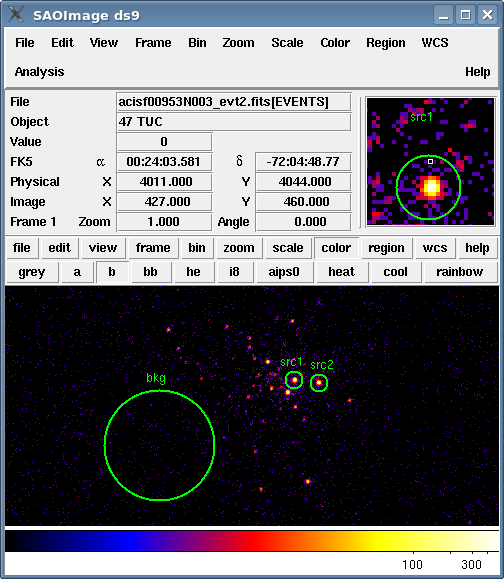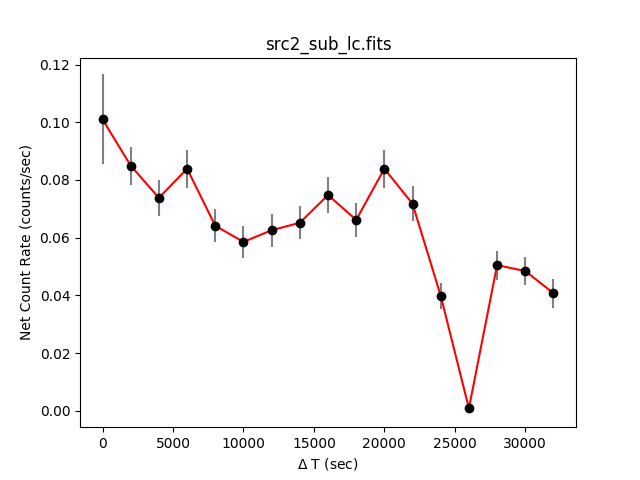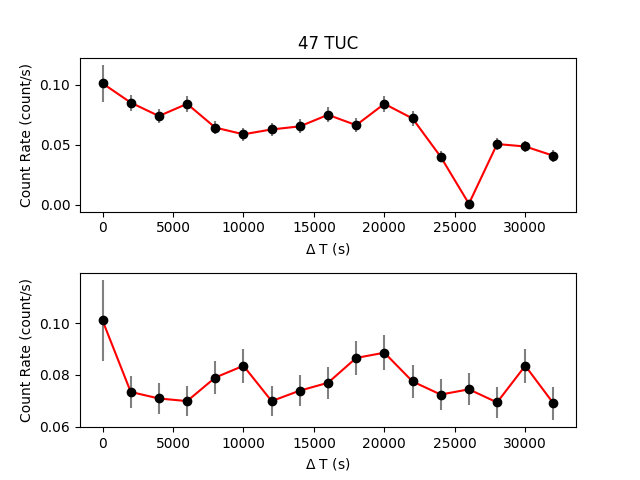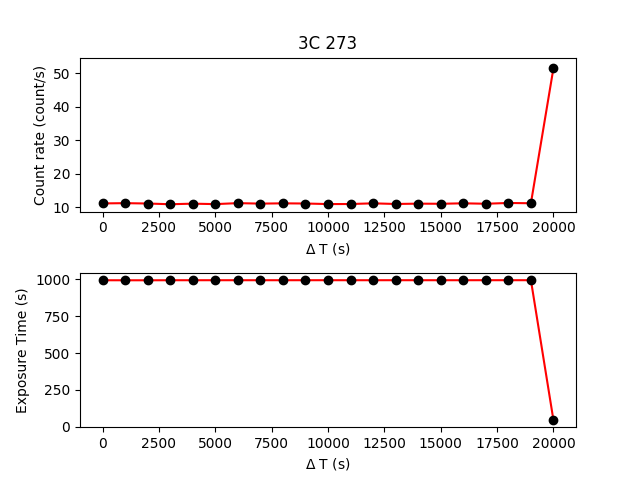# Basic Lightcurves## Overview

#### Synopsis:

A simple lightcurve from a point source in ACIS data can be used to get an idea of the variability of the source or to look for background flares that should be filtered out. The process for HRC is similar, but requires accounting for the Dead Time Factor (DTF). The CIAO tool dmextract is used in this thread as it accurately applies good time interval (GTI) information when creating lightcurves.

#### Purpose:

To create lightcurves for use in a variety of analyses.

Last Update: 2 Apr 2019 - Update to use matplotlib to plot.

## Get Started

Download the sample data: 953 (ACIS-I, 47 Tuc); 461 (HRC-I, 3C 273)

`unix% download_chandra_obsid 953,461 evt2,dtf`Early dataset

The data used in this thread was taken very early in the Chandra mission. It was not included in the bulk reprocessing metioned in the Watchout page. The keywords required for analysis with CIAO need to be added by running chandra_repro or r4_header_update.

## ACIS Lightcurves

The most common lightcurve is made from a point source observed with the ACIS detector. This may be done to get an idea of the variability of the source or to help identify periods of high background.

To begin, we define the regions - two source and one background - which will be used to create the lightcurves. For instructions on how to create regions in ds9, see the Using CIAO Region Files thread. The regions used in this example are shown in Figure 1.

### Figure 1: Image of 47 Tuc with extraction regions### Figure 1: Image of 47 Tuc with extraction regions

The source and background regions for creating the lightcurves.

```unix% cat src1.reg
# Region file format: CIAO version 1.0
circle(4010.5,4037.5,8)

unix% cat src2.reg
# Region file format: CIAO version 1.0
circle(4035.5,4034.5,8)

unix% cat bkg.reg
# Region file format: CIAO version 1.0
circle(3875.5,3972,54.5)
```

### Determine which chips are being used

dmextract uses a ccd_id filter on the input file ensure that the proper GTIs are used. Use dmstat to determine the correct chip:

```unix% punlearn dmstat
unix% dmstat "acisf00953N004_evt2.fits[sky=region(src1.reg)][cols ccd_id]"
ccd_id
min:        3             @:        1
max:        3             @:        1
mean:        3
sigma:        0
sum:        7284
good:        2428
null:        0

unix% dmstat "acisf00953N004_evt2.fits[sky=region(src2.reg)][cols ccd_id]"
ccd_id
min:        3             @:        1
max:        3             @:        1
mean:        3
sigma:        0
sum:        5850
good:        1950
null:        0

unix% dmstat "acisf00953N004_evt2.fits[sky=region(bkg.reg)][cols ccd_id]"
ccd_id
min:        3             @:        1
max:        3             @:        1
mean:        3
sigma:        0
sum:        663
good:        221
null:        0
```

The regions with which we are working are all located on chip 3 (ACIS-I3); see Figure 6.1 of the POG for an illustration of the focal plane)

### Create a background-subtracted lightcurve

First we extract a background-subtracted lightcurve for "src2":

```unix% punlearn dmextract
unix% pset dmextract infile="acisf00953N004_evt2.fits[ccd_id=3,sky=region(src2.reg)][bin time=::2000]"
unix% pset dmextract outfile="src2_sub_lc.fits"
unix% pset dmextract bkg="acisf00953N004_evt2.fits[ccd_id=3,sky=region(bkg.reg)]"
unix% pset dmextract opt="ltc1"
unix% dmextract
Input event file  (acisf00953N004_evt2.fits[ccd_id=3,sky=region(src2.reg)][bin time=::2000]):
Enter output file name (src2_sub_lc.fits):
```

You can check the parameter file that was used with plist dmextract.

The contents of the dmextract output can be shown with dmlist

```unix% dmlist src2_sub_lc.fits cols

--------------------------------------------------------------------------------
Columns for Table Block LIGHTCURVE
--------------------------------------------------------------------------------

ColNo  Name                 Unit        Type             Range
1   TIME_BIN             channel      Int4           1:17                 S/C TT corresponding to mid-exposure
2   TIME_MIN             s            Real8          69583184.1139039993: 69616983.4901389927 Minimum Value in Bin
3   TIME                 s            Real8          69583184.1139039993: 69616983.4901389927 S/C TT corresponding to mid-exposure
4   TIME_MAX             s            Real8          69583184.1139039993: 69616983.4901389927 Maximum Value in Bin
5   COUNTS               count        Int4           -                    Counts
6   STAT_ERR             count        Real8          0:+Inf               Statistical error
7   AREA                 pixel**2     Real8          -Inf:+Inf            Area of extraction
8   EXPOSURE             s            Real8          -Inf:+Inf            Time per interval
9   COUNT_RATE           count/s      Real8          0:+Inf               Rate
10   COUNT_RATE_ERR       count/s      Real8          0:+Inf               Rate Error
11   BG_COUNTS            count        Real8          -Inf:+Inf            Background Counts
12   BG_ERR               count        Real8          -Inf:+Inf            Error on Background counts
13   BG_AREA              pixel**2     Real8          -Inf:+Inf            Background Area of Extraction
14   BG_EXPOSURE          s            Real8          -Inf:+Inf            Exposure time of background file
15   BG_RATE              count/s      Real8          -Inf:+Inf            Background Rate
16   NORM_BG_COUNTS       count        Real8          -Inf:+Inf            Background Counts
17   NORM_BG_ERR          count        Real8          -Inf:+Inf            Error on Background counts
18   NET_COUNTS           count        Real8          -Inf:+Inf            Net Counts
19   NET_ERR              count        Real8          -Inf:+Inf            Error on Net Counts
20   NET_RATE             count/s      Real8          -Inf:+Inf            Net Count Rate
21   ERR_RATE             count/s      Real8          -Inf:+Inf            Error Rate

--------------------------------------------------------------------------------
World Coord Transforms for Columns in Table Block LIGHTCURVE
--------------------------------------------------------------------------------

ColNo    Name
2:    DT_MIN               = +0 [s] +1.0 * (TIME_MIN  -69584184.113904)
3:    DT                   = +0 [s] +1.0 * (TIME  -69584184.113904)
4:    DT_MAX               = +0 [s] +1.0 * (TIME_MAX  -69584184.113904)
```

The output file contains various columns including the time bin boundaries, source and background counts, area, as well as exposure times and net count rates. In addition, the TIME column has a coordinate system attached to it that provides the time relative to the start of the observation, DT.

The lightcurve may be plotted using matplotlib

```unix% python

>>> import matplotlib.pylab as plt
>>>
>>> dt = tab.get_column("dt").values
>>> rate = tab.get_column("net_rate").values
>>> erate = tab.get_column("err_rate").values
>>>
>>> plt.errorbar(dt, rate, yerr=erate, marker="o", color="red", mfc="black",mec="black", ecolor="grey")
>>> plt.xlabel("\$\Delta\$ T (sec)")
>>> plt.ylabel("Net Count Rate (counts/sec)")
>>> plt.title("src2_sub_lc.fits")
```

This lightcurve is shown in Figure 2. There is a significant drop in count rate near 26000 seconds into the observation. This information is used again in the next section.

### Figure 2: Background-subtracted lightcurve of src2### Figure 2: Background-subtracted lightcurve of src2

There is a significant drop in count rate near 26000 seconds into the observations.

Examining the lightcurve with dmlist shows the same results:

```unix% % dmlist "src2_sub_lc.fits[cols dt,time,count_rate]" data

--------------------------------------------------------------------------------
Data for Table Block LIGHTCURVE
--------------------------------------------------------------------------------

ROW    DT                   TIME                 COUNT_RATE

1                    0  69584184.1139039993     0.10108994807374
2               2000.0  69586184.1139039993           0.08507730
3               4000.0  69588184.1139039993         0.0739362250
4               6000.0  69590184.1139039993         0.0840644750
5               8000.0  69592184.1139039993        0.06431438750
6              10000.0  69594184.1139039993          0.058743850
7              12000.0  69596184.1139039993          0.062795150
8              14000.0  69598184.1139039993        0.06532721250
9              16000.0  69600184.1139039993          0.074949050
10              18000.0  69602184.1139039993        0.06634003750
11              20000.0  69604184.1139039993         0.0840644750
12              22000.0  69606184.1139039993         0.0719105750
13              24000.0  69608184.1139039993        0.04000658750
14              26000.0  69610184.1139039993         0.0010128250
15              28000.0  69612184.1139039993          0.050641250
16              30000.0  69614184.1139039993           0.04861560
17              32000.0  69616184.1139039993     0.04089879081378
```

### Looking for variability

It is also valuable to compare the lightcurves of sources in the same field when looking for variation. Here we extract a lightcurve for each of the "src1" and "src2" regions (intentionally including the background counts):

```unix% punlearn dmextract
unix% dmextract outfile="curve_1.fits" opt="ltc1" \
infile="acisf00953N004_evt2.fits[ccd_id=3,sky=region(src1.reg)][bin time=::2000]"

unix% dmextract outfile="curve_2.fits" opt="ltc1" \
infile="acisf00953N004_evt2.fits[ccd_id=3,sky=region(src2.reg)][bin time=::2000]"
```

The lightcurves can be plotted together:

```unix% python

>>> import matplotlib.pylab as plt
>>>
>>> plt.subplots(2,1,sharex="col")
>>> plt.subplot(2,1,2)
>>>
>>> dt = tab.get_column("dt")
>>> rate = tab.get_column("count_rate")
>>> erate = tab.get_column("count_rate_err")
>>> plt.errorbar(dt.values, rate.values, yerr=erate.values,
marker="o", color="red", mfc="black",mec="black", ecolor="grey")
>>> plt.xlabel(r"\$\Delta\$ T (s)")
>>> plt.ylabel("Count Rate (count/s)")
>>>
>>> plt.subplot(2,1,1)
>>> dt = tab.get_column("dt")
>>> rate = tab.get_column("count_rate")
>>> erate = tab.get_column("count_rate_err")
>>> plt.errorbar(dt.values, rate.values, yerr=erate.values,
marker="o", color="red", mfc="black",mec="black", ecolor="grey")
>>> plt.xlabel(r"\$\Delta\$ T (s)")
>>> plt.ylabel("Count Rate (count/s)")
>>> plt.title("47 TUC")
```

These commands produce Figure 3.

### Figure 3: Comparing the lightcurves### Figure 3: Comparing the lightcurves

The top plot contains lightcurve "src1" and the bottom plot contains lightcurve "src2". The x-axis values (time) for the two plots are aligned.

The results can also be examined with dmlist:

```unix% dmlist "curve_1.fits[cols time,count_rate]" data
...
13  69608184.1139039993        0.07241698750
14  69610184.1139039993        0.07444263750
15  69612184.1139039993        0.06937851250
16  69614184.1139039993        0.08355806250
17  69616184.1139039993     0.06891210431098

unix% dmlist "curve_2.fits[cols time,count_rate]" data
...
13  69608184.1139039993        0.04000658750
14  69610184.1139039993         0.0010128250
15  69612184.1139039993          0.050641250
16  69614184.1139039993           0.04861560
17  69616184.1139039993     0.04085938928173
```

Comparing this to the lightcurve data from the previous section proves two things:

1. This is not an instrumental effect, since it shows up in curve_2.fits but not curve_1.fits. An instrumental feature would appear in both sources, as they are close together and on the same chip.

2. This is not a background feature, since it is present in both the subtracted (src2_sub_lc.fits) and unsubtracted (curve_2.fits) lightcurves.

It is highly likely, therefore, that the dip in count rate is an indication of a variable star. In the case of 47 Tuc, this is due to a binary system; see the Chandra Photo Album entry for 47 Tucanae for more information.

### High background levels

The technique for identifying periods of high background from a lightcurve - and subsequently filtering them out - is explained in detail in the Filtering Lightcurves thread.

## HRC Lightcurves

The proper method of creating an HRC lightcurve requires accounting for the Dead Time Factor (DTF). The DTF describes the detector's deviation from the standard detection efficiency. This time-dependent change is due to the physical effect of an event striking the micro-channel plate, as illustrated in the MCP Principles page. The DTF is evaluated roughly every 2 seconds and the data are stored in the "dtf1.fits" file. The average DTF value within the time bin is used by dmextract to correct the exposure time and count rate in the lightcurve. Addition information about dead times can be found in the Computing Average HRC Dead Time Corrections thread.

The source region for this example has been saved in the file hrc_src.reg. Again, for instructions on how to create regions in ds9, see the Using CIAO Region Files thread.

```unix% cat hrc_src.reg
# Region file format: CIAO version 1.0
circle(16478,16292,19.5)

unix% punlearn dmextract
unix% pset dmextract infile="hrcf00461N005_evt2.fits[sky=region(hrc_src.reg)][bin time=64938947.367:64959159.548:1000]"
unix% pset dmextract outfile=hrc_lc.fits
unix% pset dmextract opt=ltc1
unix% pset dmextract exp=hrcf00461_001N004_dtf1.fits
unix% dmextract
Input event file  (hrcf00461N005_evt2.fits[sky=region(hrc_src.reg)][bin time=64938947.367:64959159.548:1000]):
Enter output file name (hrc_lc.fits):
```

You can check the parameter file that was used with plist dmextract.

Plotting the lightcurve:

```unix% python

>>> import matplotlib.pylab as plt
>>>
>>> plt.subplots(2,1,sharex="col")
>>>
>>> plt.subplot(2,1,1)
>>> dt = tab.get_column("dt")
>>> rate = tab.get_column("count_rate")
>>> erate = tab.get_column("count_rate_err")
>>> plt.errorbar(dt.values, rate.values, yerr=erate.values,
marker="o", color="red", mfc="black",mec="black", ecolor="grey")
>>> plt.xlabel(r"\$\Delta\$ T (s)")
>>> plt.ylabel("Count rate (count/s)")
>>> plt.title("3C 273")
>>>
>>> plt.subplot(2,1,2)
>>> ee = tab.get_column("EXPOSURE")
>>> plt.plot(dt.values, ee.values,
marker="o", color="red", mfc="black",mec="black")
>>> plt.xlabel(r"\$\Delta\$ T (s)")
>>> plt.ylabel("Exposure Time (s)")
```

creates Figure 4.

### Figure 4: HRC lightcurve### Figure 4: HRC lightcurve

The Dead Time Factor (DTF) was included when creating this lightcurve. Note that the final time bin has a very low exposure value (bottom plot). This leads to an anomolously high count rate (top plot).

Examine the lightcurve with dmlist:

```unix% dmlist "hrc_lc.fits[cols time,count_rate]" data

--------------------------------------------------------------------------------
Data for Table Block LIGHTCURVE
--------------------------------------------------------------------------------

ROW    TIME                 COUNT_RATE

1  64939447.3669999987        11.0732902274
2  64940447.3669999987        11.1508118948
3  64941447.3669999987        11.0442979298
4  64942447.3669999987        10.8239338975
5  64943447.3669999987        11.0010478186
6  64944447.3669999987        10.8625693020
7  64945447.3669999987        11.1579373574
8  64946447.3669999987        11.0144558786
9  64947447.3669999987        11.0962446993
10  64948447.3669999987        11.0444782872
11  64949447.3669999987        10.8780703573
12  64950447.3669999987        10.8946114585
13  64951447.3669999987        11.0979602670
14  64952447.3669999987        10.9051273434
15  64953447.3669999987        11.0032931023
16  64954447.3669999987        10.9659395796
17  64955447.3669999987        11.1047841342
18  64956447.3669999987        10.9752825787
19  64957447.3669999987        11.2070096507
20  64958447.3669999987        11.1219813983
21  64959447.3669999987        51.5457065863
```

## Caveats

There are a number of subtleties that it is important to be aware of when using lightcurves for timing analysis. These issues are described in the Timing Analysis with Lightcurves why topic; please read that document before continuing with the analysis.

```

#--------------------------------------------------------------------
#
# DMEXTRACT -- extract columns or counts from an event list
#
#--------------------------------------------------------------------
infile = acisf00953N004_evt2.fits[ccd_id=3,sky=region(src2.reg)][bin time=::2000] Input event file
outfile = src2_sub_lc.fits Enter output file name
(bkg = acisf00953N004_evt2.fits[ccd_id=3,sky=region(bkg.reg)]) Background
region file or fixed background (counts/pixel/s) subtraction
(error = gaussian)        Method for error determination(poisson|gaussian|<variance file>)
(bkgerror = gaussian)        Method for background error determination(poisson|gaussian|<variance file>)
(bkgnorm = 1.0)             Background normalization
(exp = )                Exposure map image file
(bkgexp = )                Background exposure map image file
(sys_err = 0)               Fixed systematic error value for SYS_ERR keyword
(opt = ltc1)            Output file type: pha1
(defaults = \${ASCDS_CALIB}/cxo.mdb -> /soft/ciao/data/cxo.mdb) Instrument defaults file
(wmap = )                WMAP filter/binning (e.g. det=8 or default)
(clobber = no)              OK to overwrite existing output file(s)?
(verbose = 0)               Verbosity level
(mode = ql)
```

```

#--------------------------------------------------------------------
#
# DMEXTRACT -- extract columns or counts from an event list
#
#--------------------------------------------------------------------
infile = hrcf00461N005_evt2.fits[sky=region(hrc_src.reg)][bin time=64938947.367:64959159.548:1000] Input event file
outfile = hrc_lc.fits      Enter output file name
(bkg = )                Background region file or fixed background (counts/pixel/s) subtraction
(error = gaussian)        Method for error determination(poisson|gaussian|<variance file>)
(bkgerror = gaussian)        Method for background error determination(poisson|gaussian|<variance file>)
(bkgnorm = 1.0)             Background normalization
(exp = hrcf00461_001N004_dtf1.fits) Exposure map image file
(bkgexp = )                Background exposure map image file
(sys_err = 0)               Fixed systematic error value for SYS_ERR keyword
(opt = ltc1)            Output file type
(defaults = \${ASCDS_CALIB}/cxo.mdb -> /soft/ciao/data/cxo.mdb) Instrument defaults file
(wmap = )                WMAP filter/binning (e.g. det=8 or default)
(clobber = no)              OK to overwrite existing output file(s)?
(verbose = 0)               Verbosity level
(mode = ql)
```